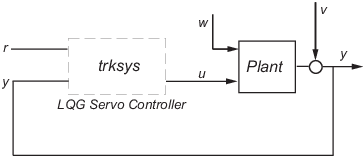## Design an LQG Servo Controller

This example shows you how to design a servo controller for the following system.The plant has three states (x), two control inputs (u), two random inputs (w), one output (y), measurement noise for the output (v), and the following state and measurement equations:

`$\begin{array}{l}\stackrel{˙}{x}=Ax+Bu+Gw\\ y=Cx+Du+Hw+v\end{array}$`

where

`$\begin{array}{l}A=\left[\begin{array}{ccc}0& 1& 0\\ 0& 0& 1\\ 1& 0& 0\end{array}\right]\text{ }\text{ }B=\left[\begin{array}{cc}0.3& 1\\ 0& 1\\ -0.3& 0.9\end{array}\right]\text{ }\text{ }G=\left[\begin{array}{cc}-0.7& 1.12\\ -1.17& 1\\ 0.14& 1.5\end{array}\right]\\ \\ C=\left[\begin{array}{ccc}1.9& 1.3& 1\end{array}\right]\text{ }D=\left[\begin{array}{cc}0.53& -0.61\end{array}\right]\text{ }H=\left[\begin{array}{cc}-1.2& -0.89\end{array}\right]\end{array}$`

The system has the following noise covariance data:

`$\begin{array}{l}{Q}_{n}=E\left(w{w}^{T}\right)=\left[\begin{array}{cc}4& 2\\ 2& 1\end{array}\right]\text{ }\text{ }\\ Rn=E\left(v{v}^{T}\right)=0.7\end{array}$`

Use the following cost function to define the tradeoff between tracker performance and control effort:

`$J\left(u\right)={\int }_{0}^{\infty }\left(0.1{x}^{T}x+{x}_{i}^{2}+{u}^{T}\left[\begin{array}{cc}1& 0\\ 0& 2\end{array}\right]u\right)dt$`

To design an LQG servo controller for this system:

1. Create the state space system by typing the following in the MATLAB Command Window:

```A = [0 1 0;0 0 1;1 0 0]; B = [0.3 1;0 1;-0.3 0.9]; G = [-0.7 1.12; -1.17 1; .14 1.5]; C = [1.9 1.3 1]; D = [0.53 -0.61]; H = [-1.2 -0.89]; sys = ss(A,[B G],C,[D H]);```

2. Construct the optimal state-feedback gain using the given cost function by typing the following commands:

```nx = 3; %Number of states ny = 1; %Number of outputs Q = blkdiag(0.1*eye(nx),eye(ny)); R = [1 0;0 2]; K = lqi(ss(A,B,C,D),Q,R); ```

3. Construct the Kalman state estimator using the given noise covariance data by typing the following commands:

```Qn = [4 2;2 1]; Rn = 0.7; kest = kalman(sys,Qn,Rn); ```

4. Connect the Kalman state estimator and the optimal state-feedback gain to form the LQG servo controller by typing the following command:

`trksys = lqgtrack(kest,K)`
This command returns the following LQG servo controller:
```>> trksys = lqgtrack(kest,K) a = x1_e x2_e x3_e xi1 x1_e -2.373 -1.062 -1.649 0.772 x2_e -3.443 -2.876 -1.335 0.6351 x3_e -1.963 -2.483 -2.043 0.4049 xi1 0 0 0 0 b = r1 y1 x1_e 0 0.2849 x2_e 0 0.7727 x3_e 0 0.7058 xi1 1 -1 c = x1_e x2_e x3_e xi1 u1 -0.5388 -0.4173 -0.2481 0.5578 u2 -1.492 -1.388 -1.131 0.5869 d = r1 y1 u1 0 0 u2 0 0 Input groups: Name Channels Setpoint 1 Measurement 2 Output groups: Name Channels Controls 1,2 Continuous-time model.```